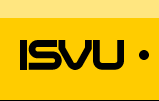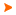Podaci o VU Početna stranicaArhiva nastavnih programaArhiva ak. kalendaraMechanical Design Optimization
 Abbreviation: OMK Load: 30(L) + 15(E) + 0(LE) + 0(CE) + 0(PEE) + 0(FE) + 0(S) + 0(DE) + 0(P) + 0(FLE) + 0()
 Lecturers in charge: izv. prof. dr. sc. Andrej Jokić Lecturers: doc. dr. sc. Matija Hoić ( Exercises ) izv. prof. dr. sc. Andrej Jokić ( Exercises ) Course description: Course objectives: Familiarize with methods of optimal design of constructions and their elements. Learn to create an appropriate mathematical model of the design problem, to formulate the optimization problem and finally to use numerical optimization techniques to solve the problem. Acquire the fundamental knowledge of global optimization.Enrolment requirements and required entry competences for the course: Student responsibilities: attendance to lectures and exercises, individual solving and presentation of examples, pass preliminary exam.Grading and evaluation of student work over the course of instruction and at a final exam: activity and attendance during classes (lectures and exercises) 20%, individual examples solving 40%, preliminary exam 40%.Methods of monitoring quality that ensure acquisition of exit competences: Upon successful completion of the course, students will be able to (learning outcomes): apply rules of the mathematical model scheme to problems of optimal design of the mechanical constructions; use the classical optimization methods (Lagrange multipliers, optimality conditions) for solving problems with restrictions; create mathematical optimization models for joints calculation, design and analysis of constructions and for optimal synthesis of the planar mechanisms; apply appropriate numerical methods and algorithms in the solution to problems of nonlinear and linear optimization; explain specifics and solution methods in global optimization problems; evaluate optimization results.Lectures 1. Introduction. Conventional and optimum design process. Design space, variables, objective function, design constraints. Examples.2. The formulation rules of mathematical models. Graphical optimization methods in 2D design space. Examples.3. Unimodal optimization problems. The methods of solution and algorithms. Onedimensional minimization, Fibonacci and Golden section method.4. Unconstrained and constrained design space. The method of Lagrange multipliers.5. Optimality conditions. Lagrange multipliers example.6. Constrained optimization based on the direct search methods. Flexible polyhedron (NelderMead) method.7. Algorithm of NelderMead method. Examples. Sequential unconstrained optimization. Penalty function method.8. Introduction to linear programming. The methods, application, algorithms.9. Multiobjective optimization. Pareto optimum. Examples.10. Practical optimization examples of structures. Optimum design of construction elements (girders, bars).11. Applying the optimization methods in calculation of statically undetermined structures. Examples12. Optimal synthesis of planar linkages. Chebyshev polynomials. Minimization of the structural error.13. The formulation rules to optimal design of mechanism. Optimal synthesis of planar linkages for path generation. Examples: Chebyshev, Evans and Watt"s, mechanism.14. Optimal design of structures. Minimum weight, dimensional and structural truss optimization. Fullystressed design.15. Global optimization, application and algorithms. The method of differential evolution. Examples test functions.Exercises 1. Illustration Examples. Optimum design problem formulation.2. Illustration Examples. Graphical optimization.3. Illustration Examples.4. Illustration Examples. Lagrange multipliers.5. Illustration Examples. Method of Lagrange multipliers.6. Illustration Examples. Flexible polyhedron (simplex) method.7. Illustration Examples.8. Exercises set problems to be solved. Individual solving of the problem. Consultation.9. Illustration Examples. Individual solving of the problem. Consultation.10. Illustration Examples. Individual solving of the problem. Consultation.11. Illustration Examples. Individual solving of the problem. Consultation.12. Illustration Examples. Individual solving of the problem. Consultation.13. Illustration Examples. Individual solving of the problem. Consultation.14. Illustration Examples. Individual solving of the problem. Consultation.15. Presentation of solved problem. Preliminary exam.
 Lecture languages: hr
 Compulsory literature: 1. D. Ščap, Optimiranje mehaničkih konstrukcija, udžbenik u radu (raspoloživo za studente). 2. J. S. Arora, Introduction to optimum design, McGraw-Hill, New York, 2004. 3. K.V. Price, R.M. Storn, J.A. Lampinen, Differential Evolution, A Practical Approach to Global Optimization, Springer-Verlag, Berlin Heidelberg, 2005.
 Recommended literature: - - -Legend L - Lectures FLE - Practical foreign language exercises - E - Exercises LE - Laboratory exercises CE - Project laboratory PEE - Physical education excercises FE - Field exercises S - Seminar DE - Design exercises P - Practicum * - Not graded| Početna stranica | Visoka učilišta | Korisničke stranice | | Na vrh | Copyright (c) 2006. Ministarstva znanosti, obrazovanja i športa. Sva prava zadržana. Programska podrška (c) 2006. Fakultet elektrotehnike i računarstva. Oblikovanje(c) 2006. Listopad Web Studio. Posljednja izmjena 2019-06-07